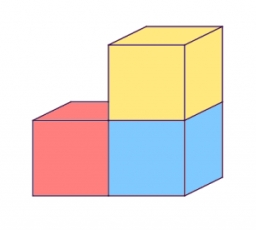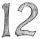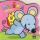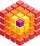# The wooden

The wooden block measures 12 cm, 24 cm, and 30 cm. Peter wants to cut it into several identical cubes. At least how many cubes can he get?

x =  40

### Step-by-step explanation:Did you find an error or inaccuracy? Feel free to write us. Thank you!Tips to related online calculators
Do you want to calculate greatest common divisor two or more numbers?
Tip: Our volume units converter will help you with the conversion of volume units.

## Related math problems and questions:

• Identical cubesFrom the smallest number of identical cubes whose edge length is expressed by a natural number, can we build a block with dimensions 12dm x 16dm x 20dm?
• CubesCarol with cut bar 12 cm x 12 cm x 135 cm to the cubes. Find the sum of all the surfaces of the resulting cubes.
• The cubeThe cube has an edge of 25 cm. We cut it into small cubes of 5 cm long side. How many of these little ones left when we build a new cube of 20 cm in length?
• Gcd and lcmCalculate the greatest common divisor and the least common multiple of numbers. a) 16 and 18 b) 24 and 22 c) 45 and 60 d) 36 and 30
• Cubes into cuboidHow many 12 centimeter cubes fit into the block (cuboid) with 6dm, 8.4dm, and 4.8?
• Wooden blockA cuboid-shaped wooden block has 6 cm length, 4 cm breadth, and 1 cm height. Two faces measuring 4 cm x 1 cm are colored in black. Two faces measuring 6 cm x 1 cm are colored in red. Two faces measuring 6 cm x 4 cm are colored in green. The block is divid
• Bricks pyramidHow many 50cm x 32cm x 30cm brick needed to built a 272m x 272m x 278m pyramid?
• Water blockA block with a 50 cm2 base is filled with water 5 cm under the edge. How many can sugar cubes with 2 cm edge be thrown into a container that overflow water?
• Mouse HryzkaMouse Hryzka found 27 identical cubes of cheese. She first put in a large cube out of them and then waited for a while before the cheese cubes stuck together. Then from every wall of the big cube she will eats the middle cube. Then she also eats the cube
• QuotientFind quotient before the bracket - the largest divisor 51 a + 34 b + 68 121y-99z-33
• Cube cornersThe wooden cube with edge 64 cm was cut in 3 corners of cube with edge 4 cm. How many cubes of edge 4 cm can be even cut?
• For thinkingsThe glass cube dive into the aquarium, which has a length of 25 cm, width 20 cm and height of 30 cm. Aquarium water rises by 2 cm. a) What is the volume of a cube? b) How many centimeters measure its edge?
• Mike choseMike chose 4 identical cubes, 3 identical prisms and 2 identical cylinders from the kit. The edge of the cube is 3 cm long. The prism has two dimensions the same as the cube, its third dimension is 2 times longer. The diameter of the base of the cylinder
• Cuboid aquariumCuboid 25 times 30 cm. How long is third side if cuboid contains 30 liters of water?
• Into boxHow many cubes with an edge of 2.5 cm fit into a box measuring 11.6 cm; 8.9 cm and 13.75 cm?
• Cloth / textileWe have cloth measure 16 square meters. How many 20 cm by 20 cm by 8 cm bags you can make? Assume bag is a cuboid without one top base.
• Lcm = 22 + gcdThe least common multiple of two numbers is 22 more than their greatest common divisor. Find these numbers.